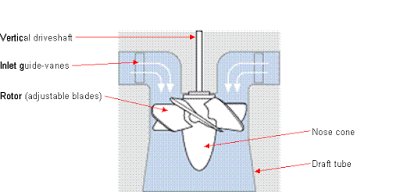# What is Turbine? What Are Main Types of Turbines?

Sharing is Caring :)-

Today we will learn about what is turbine and types of turbines used in mechanical industries. We have heard many time the word “turbine”, but most of us don’t know more about It. Turbine is a device which convert kinetic energy of fluid into mechanical energy. The fluid may be either water or gas or steam according to the availability. It is mainly used in power plant industries to generate electric power. It can be categorized into three types. The first on is known as hydraulic turbine, second one is gas turbine and the last one is steam turbine.

Hydraulic turbine is an hydraulic machines which convert hydraulic energy of water into mechanical energy. It installed at dam or near any large water reservoir.

Gas turbine uses compressed air or other gases to rotate the turbine and convert kinetic energy of gases into mechanical energy.

The last one is steam turbine in which first water is converted into high pressure super heated steam, which is further used to drive turbine.

## What is turbine?

A hydraulic machine which converts hydraulic energy into
mechanical energy is known as turbine. Turbine is a device which converts kinetic energy of water, air, gas or steam into mechanical energy which is further used to rotate generator shaft. Simply turbine is a rotor which has vanes at the peripheral and connected at the end of an electromagnet or well known generator shaft which causes to rotate it and generate electric power.

We know that turbine is mostly used in hydroelectric power
plants. As shown in diagram a dam is constructed across a river to store water. There
is an elevation difference between turbine and dam. Turbine and dam is connected
by a large diameter pipe called penstock which carries pressurize water from the storage reservoir to turbine. The turbine is connected to an electromagnet. When water strikes the turbine blades, it makes rotate the turbine hence electric generator which produces electric power. Turbine has different types of vanes fitted on rotter. The water is carried to tail race after worked on the
turbine.

For clear understanding watch the following video.

## Types of Turbines:

Turbine can be classified in various ways like according to direction of fluid, head at the inlet of turbine, types of fluid etc. These
are

### According to the type of energy at inlet:

1. Impulse turbine:

If a turbine uses only impulse energy of fluid, it is known as impulse turbine. The impulse energy means it uses only kinetic energy of fluid. In this type of turbine, first whole pressure energy or head converted into kinetic energy and this kinetic energy used to rotate turbine. The water
uses only its kinetic energy and the pressure of water remains constant at inlet and exit of it.

Example : Pelton Wheel

2. Reaction turbine:

If the water pressure at inlet and exit of
turbine is different or the water use sits kinetic energy as well as pressure
energy to rotate the turbine, is known as reaction turbine. When the water under
pressure flows through the turbine, some of its pressure energy converts into
kinetic energy which rotate the turbine.
Example: Francis TurbineKaplan Turbine (Reaction Turbine)

### According to the direction of flow through runner:

1. Tangential flow turbine:
If the water flows along the tangent of the runner, the
turbine is known as tangential flow turbine. Pelton wheel turbine is the
example of tangential flow turbine.
If the water flows in the radial direction through the runner,
the turbine is called radial flow turbine. If the water flows from outward to
inward, radially, the turbine is known as inward radial flow turbine and if oppose
the turbine is known as outward radial flow turbine.
3. Axial flow turbine:
If the water flows along the direction parallel to the axis
of the runner, the turbine is known as axial flow turbine. Kaplan Turbine is the
example of axial flow turbine.
4. Mixed flow turbine:
If the water flows through the runner in the radial direction
but leaves in the direction parallel to the axis of rotation of the runner, the
turbine is called mixed flow turbine. Francis is the example of mixed flow turbine.

### According to specific speed of turbine:

1. Low specific speed turbine
2. Medium specific speed turbine
3. High specific speed turbine

Also Read : Types of Gas Turbine

Today we have learnt about what is turbine and types of turbines. If you have any doubt regarding this article, ask by commenting.

Sharing is Caring :)-

### 3 thoughts on “What is Turbine? What Are Main Types of Turbines?”

•September 12, 2017 at 5:28 am

thanks for providing this awesome notes

•May 13, 2019 at 2:00 pm
•